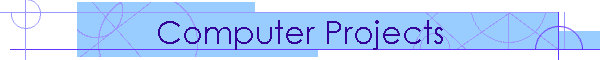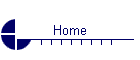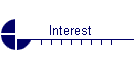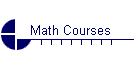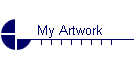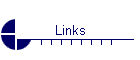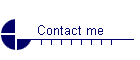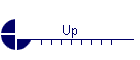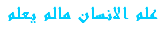Math 260 Spring 2004 (032) Computer Project 1  (NiN)      This project consists of selected problem from major exam 1 to be solved using Maple and Mathcad. Computer Project 2  (mcd)   The student ID, list, and section numbers are used to create an individual scalar 3rd-order homogeneous differential equation with constant coefficients and its initial conditions. Mathcad is used to automatically find the general solution and a particular solution satisfying the initial conditions. The student is then asked to transform the scalar equation into a system of first order equations and use Mathcad to solve the system using the eigenvalue-eigenvector method. Then, he is asked to  compare the two solutions and find their relationship. Note:  The scalar equation is designed with a characteristic equation having a root with multiplicity 3 so that the transformation will give a system with an eignvalue of multiplicity 3 and defect 2. Computer Project 2  (HTML)    This is the HTML format to view the project in case Mathcad 2000 or later is not  installed locally.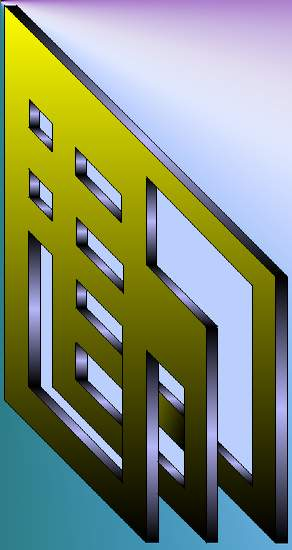A. Farhat 2003